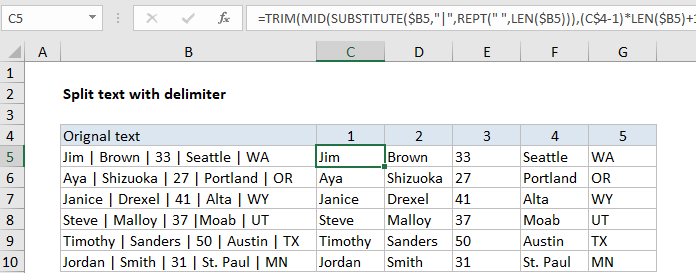## Excel Office

Excel How Tos, Tutorials, Tips & Tricks, Shortcuts

# How to split text with delimiter in Excel

To split text at an arbitrary delimiter (comma, space, pipe, etc.) you can use a formula based on the TRIM, MID, SUBSTITUTE, REPT, and LEN functions.

## Formula

```=TRIM(MID(SUBSTITUTE(A1,delim,REPT
(" ",LEN(A1))),(N-1)*LEN(A1)+1,LEN(A1)))```## Explanation

In the example shown, the formula in C5 is:

```=TRIM(MID(SUBSTITUTE(\$B5,"|",
REPT(" ",LEN(\$B5))),(C\$4-1)*
LEN(\$B5)+1,LEN(\$B5)))```

Note: references to B5 and C4 are mixed references to allow the formula to be copied across and down.

### How this formula works

The gist of this formula is to replace a given delimiter with a large number of spaces using SUBSTITUTE and REPT, then use the MID function to extract text related to the “nth occurrence” and the TRIM function to get rid of the extra space.

Also See:   How to abbreviate names or words in Excel

In this snippet, the delimiter (delim) is replaced with a number of spaces equal to the total length of the string:

`SUBSTITUTE(A1,delim,REPT(" ",LEN(A1)))`

Then the formula uses the MID function to extract the nth substring. The starting point is calculated with the code below, where N represents “nth”:

`(N-1)*LEN(A1)+1`

The total characters extracted is equal to the length of the full text string. The TRIM function then removes all extra spaces and returns just the nth string.

Also See:   TRIM function: Description, Usage, Syntax, Examples and Explanation

### Extract just one instance

Although the example is set up to extract 5 substrings from the text in column B, you can easily extract just 1 instance. For example, to extract just the 4th item (city), you could use:

```=TRIM(MID(SUBSTITUTE(B5,"|",REPT
(" ",LEN(B5))),(4-1)*LEN(B5)+1,LEN(B5)))```

### Text to Columns feature

For manual, one-off conversions, Excel has a built-in feature called “Text to Columns” that can split text in cells with a delimiter of your choice. You’ll find this feature on the the Data tab of the ribbon in the Data tools section.

Also See:   How to strip html from text or numbers in Excel